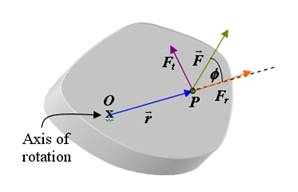## 11.7   TorqueSuppose a force is applied at point as shown in the figure above. Point is part of a rigid body with an axis of rotation going through point . Suppose the angle between the force and the position vector is . The force can be decomposed into which does not produce rotation and which does produce rotation about the origin . The component is given by

Another way to look at the torque equation is to consider the following

If more than one force is acting on a rigid body, the net torque (or resultant torque) is

Demos/Animations Examples/Checkpoints Interactive Problems
am = animation ,dm = demo, cp = checkpoint, ex = example, ip = interactive problem